// This prints the left floatting menu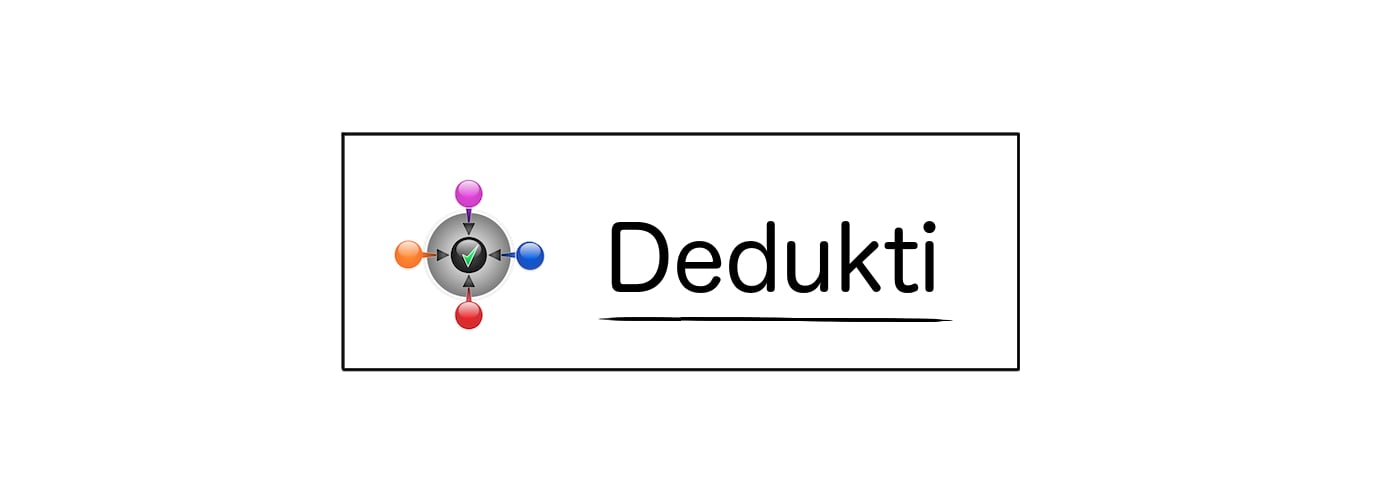### Theorembool.bool_discr

Statement

∀ x y, x = y ⇒ if x then (if y then (∀ P, P ⇒ P) else (∀ P, P)) else (if y then (∀ P, P) else (∀ P, P ⇒ P))

Main Dependencies
Theory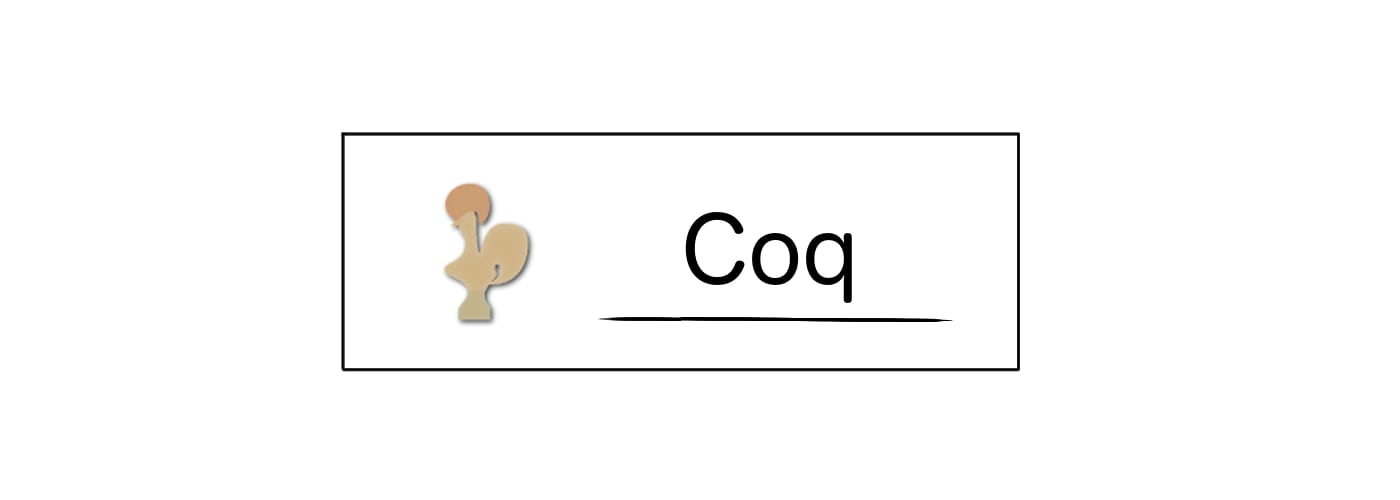Statement

Theorem bool_discr : forall (x:bool), forall (y:bool), (logic.eq (bool) x y) -> match_bool_type (Prop) (match_bool_type (Prop) (forall (P:Prop), P -> P) (forall (P:Prop), P) y) (match_bool_type (Prop) (forall (P:Prop), P) (forall (P:Prop), P -> P) y) x.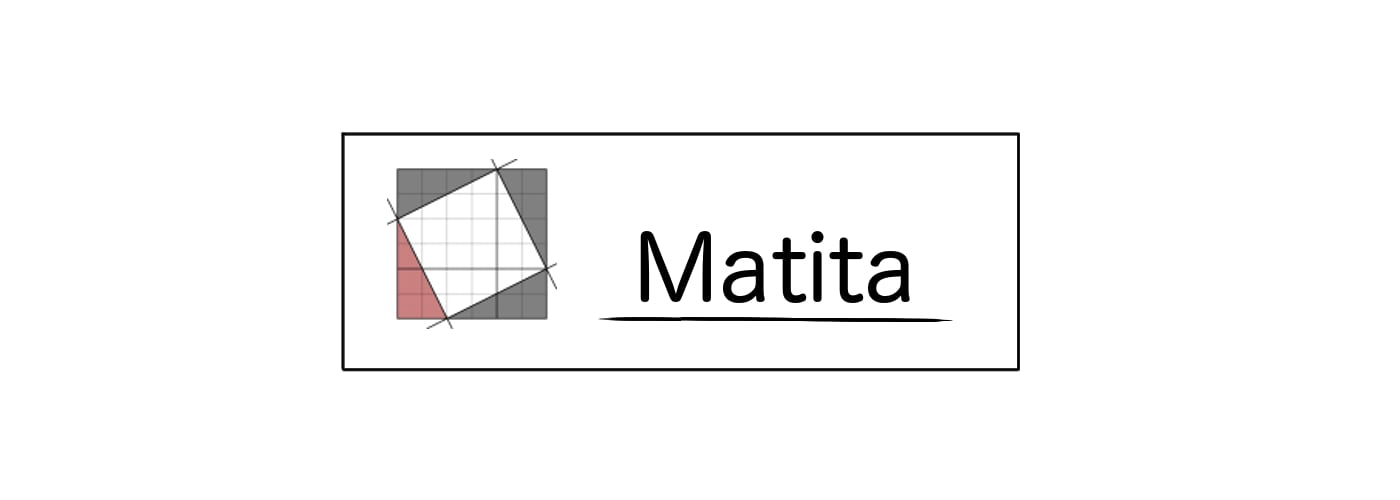Statement

theorem bool_discr : \forall (x:bool). \forall (y:bool). ((eq) (bool) x y) -> (match_bool_type) (Prop) ((match_bool_type) (Prop) (\forall (P:Prop). (P) -> P) (\forall (P:Prop). P) y) ((match_bool_type) (Prop) (\forall (P:Prop). P) (\forall (P:Prop). (P) -> P) y) x.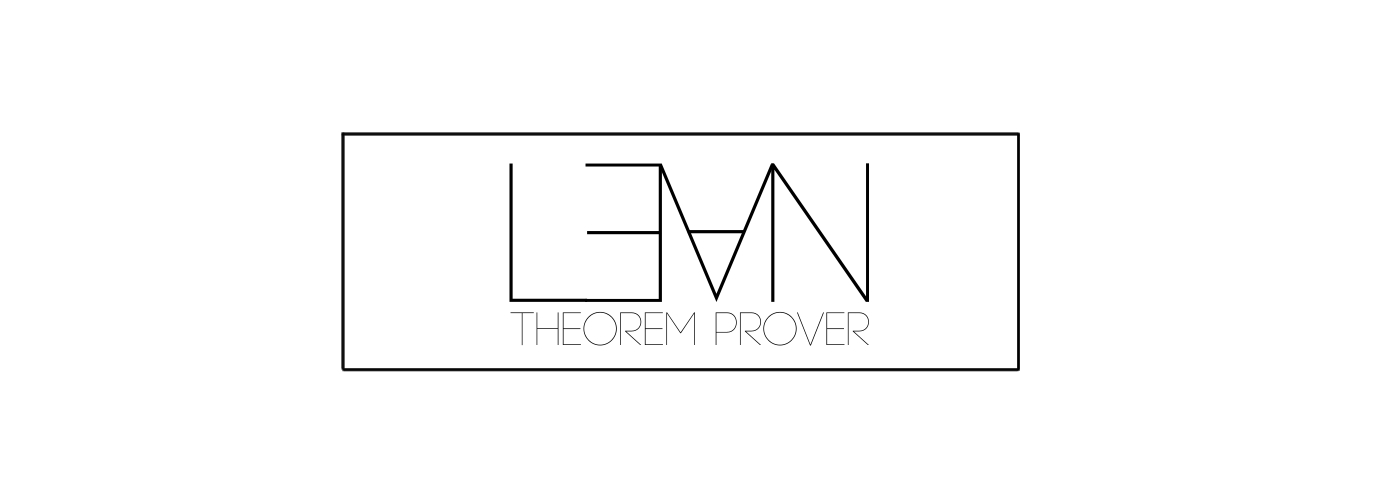Statement

theorem bool_discr : forall (x:bool.bool) , forall (y:bool.bool) , ((((logic.eq_) (bool.bool)) (x)) (y)) -> ((((bool.match_bool_type) (Prop)) (((((bool.match_bool_type) (Prop)) (forall (P:Prop) , (P) -> P)) (forall (P:Prop) , P)) (y))) (((((bool.match_bool_type) (Prop)) (forall (P:Prop) , P)) (forall (P:Prop) , (P) -> P)) (y))) (x).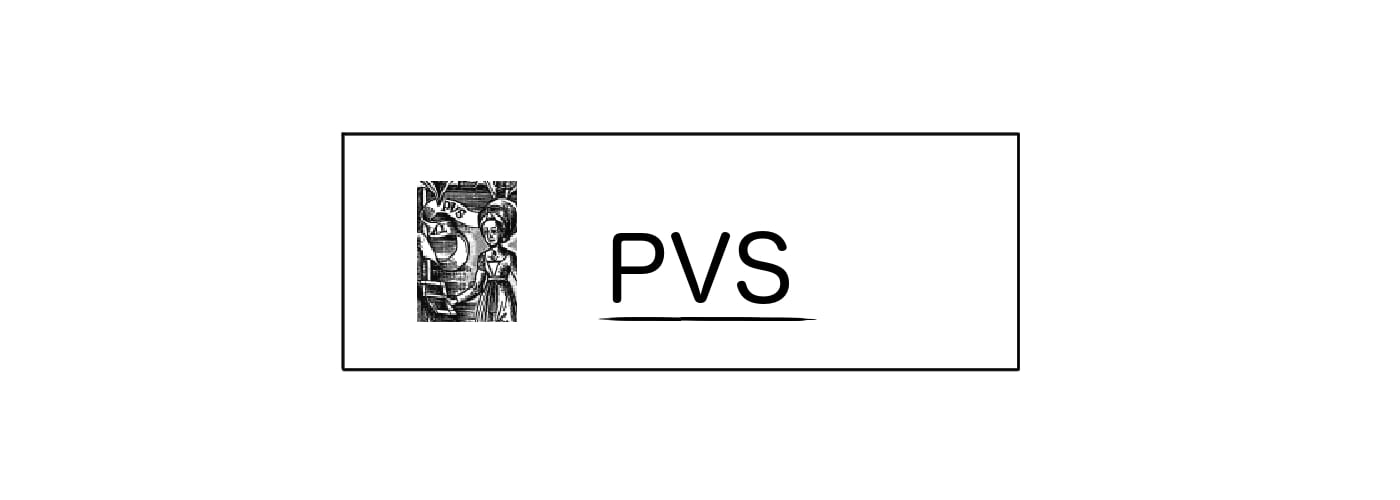Statement

bool_discr : LEMMA (FORALL(x:bool_sttfa.sttfa_bool):(FORALL(y:bool_sttfa.sttfa_bool):(logic_sttfa_th.eq[bool_sttfa.sttfa_bool](x)(y) => bool_sttfa.match_bool_type[bool](bool_sttfa.match_bool_type[bool]((FORALL(P:bool):(P => P)))((FORALL(P:bool):P))(y))(bool_sttfa.match_bool_type[bool]((FORALL(P:bool):P))((FORALL(P:bool):(P => P)))(y))(x))))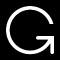Printing for OpenTheory is not working at the moment.